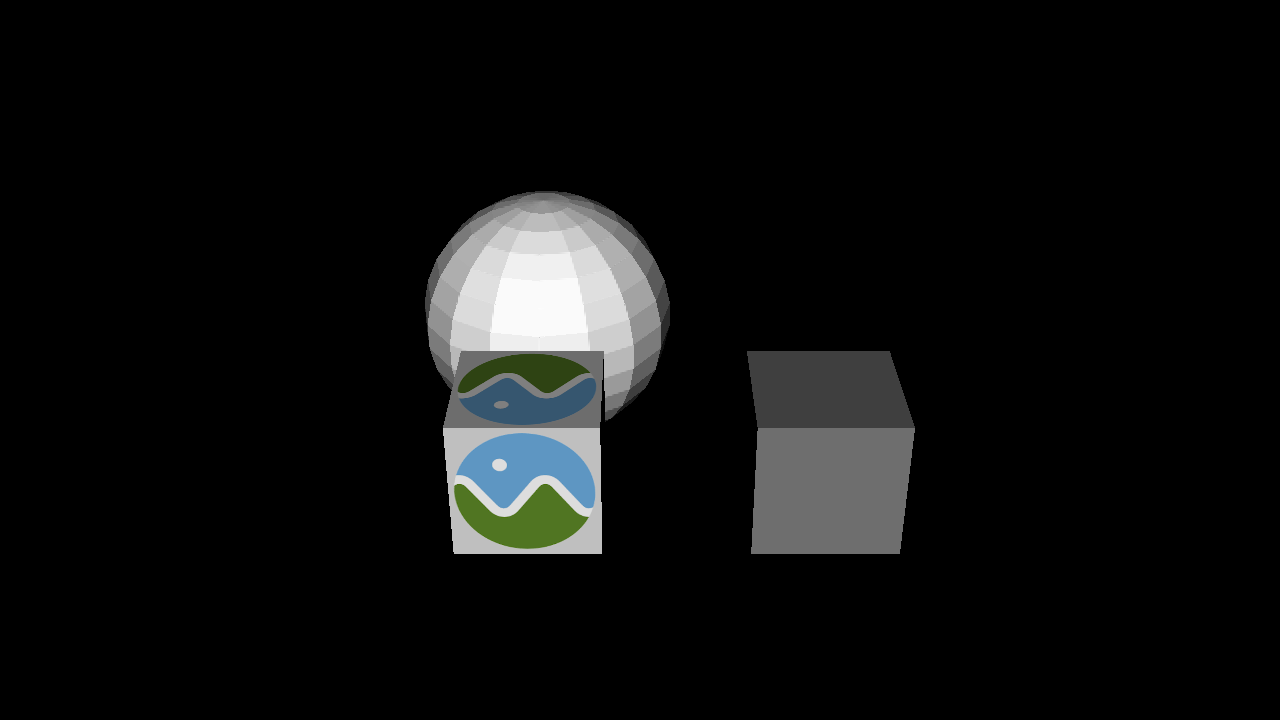# Exporting scene as a glTF file#

In this tutorial, we will show how to create a glTF file for a scene.

```import numpy as np

from fury import actor, gltf, window
```

Specifying centers and colors for actors. We will use these parameters later.

```centers = np.zeros((3, 3))
colors = np.array([1, 1, 1])
```

Create a scene.

```scene = window.Scene()
```

Creating actors and adding to scene.

```cube = actor.cube(np.add(centers, np.array([2, 0, 0])), colors=colors / 2)

sphere = actor.sphere(np.add(centers, np.array([0, 2, 0])), colors=colors)

fetch_gltf('BoxTextured', 'glTF')
gltf_obj = gltf.glTF(filename)
box_actor = gltf_obj.actors()
```

Setting camera to the scene.

```scene.set_camera(
position=(4.45, -21, 12), focal_point=(4.45, 0.0, 0.0), view_up=(0.0, 0.0, 1.0)
)
```

Exporting scene as a glTF file

```gltf.export_scene(scene, filename='viz_gltf_export.gltf')
```Reading the newly created glTF file and get actors.

```gltf_obj = gltf.glTF('viz_gltf_export.gltf')
actors = gltf_obj.actors()
```

Add all the actor from list of actors to the scene.

```scene.add(*actors)

interactive = False

if interactive:
window.show(scene, size=(1280, 720))

window.record(scene, out_path='viz_gltf_export.png', size=(1280, 720))
```Total running time of the script: ( 0 minutes 0.118 seconds)

Gallery generated by Sphinx-Gallery